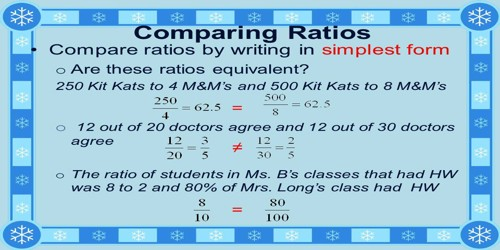Mathematic

# How to Compare Ratios?Ratios are used to compare values. In mathematics, a ratio is a relationship between two numbers indicating how many times the first number contains the second. The ratio of two like quantities a and b is the fraction a/b, which indicates how many times b is the quantity a. In other words, their ratio indicates their relative sizes.

Example: Suppose three men receive their share of profit as 4000, 3000 and 1000. Find the ratio between their profits.

Solution:

The ratio of their share (profits) is

4000 : 3000 : 1000

= 4 : 3 : 1         (divided by 1000)

To compare two ratios, follow these steps:

Step I: Make the second term of both the ratios equal.

For this, determine the LCM of the second terms of the ratios. Divide the LCM by the second term of each ratio. Multiply the numerator and the denominator of each ratio by the quotient.

Step II: Compare the first terms (numerators) of the new ratios.

Remember: A Ratio A ratio is a comparison of two numbers that can be expressed in the following three ways: Example: as a fraction: 2/4 with a colon: 2:4 with “to”: 2 to 4

Example:

Are the ratios 3 to 4 and 6:8 equal?

The ratios are equal if 3/4 = 6/8.

These are equal if their cross products are equal; that is, if 3 × 8 = 4 × 6. Since both of these products equal 24, the answer is yes, the ratios are equal.

A ratio of 1:7 is not the same as a ratio of 7:1.

Examples:

Are the ratios 7:1 and 4:81 equal? No!

7/1 > 1, but 4/81 < 1, so the ratios can’t be equal.

Are 7:14 and 36:72 equal?

Notice that 7/14 and 36/72 are both equal to 1/2, so the two ratios are equal.

Information Source: